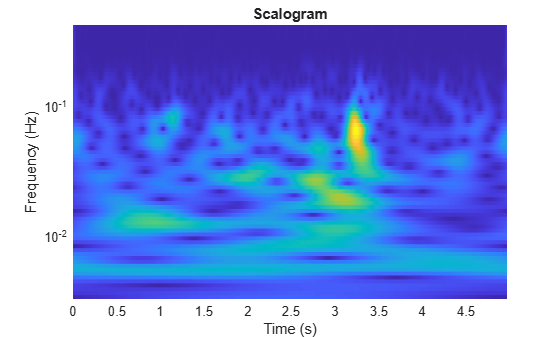# dlcwt

Deep learning continuous wavelet transform

Since R2022b

## Syntax

``cfs = dlcwt(x,psifvec,filteridx)``
``cfs = dlcwt(x,psifvec,filteridx,DataFormat=fmt)``

## Description

````cfs = dlcwt(x,psifvec,filteridx)` returns the deep learning continuous wavelet transform (CWT) of `x`. `psifvec` is a real-valued CWT filter bank, and `filteridx` is a bookkeeping matrix. `dlcwt` requires Deep Learning Toolbox™.```

example

````cfs = dlcwt(x,psifvec,filteridx,DataFormat=fmt)` specifies the data format of `x`.```

## Examples

collapse all

Load the ECG signal. The sampling frequency of the data is 180 hertz. Save the signal as a `dlarray` in `"CBT"` format.

```load wecg Fs = 180; sig = dlarray(reshape(wecg,1,1,[]),"CBT");```

Create a CWT filter bank that is compatible with the signal. Specify periodic boundary conditions.

`fb = cwtfilterbank(SignalLength=length(sig),Boundary="periodic");`

Use the `wt` object function to obtain the CWT coefficients of `wecg`. Also obtain the scaling coefficients. Concatenate the coefficients.

```[cfsFB,~,~,scalcfs] = wt(fb,wecg); allCFS = [cfsFB ; scalcfs]; whos allCFS```
``` Name Size Bytes Class Attributes allCFS 82x2048 2686976 double complex ```

Use the `cwtfilters2array` function to convert the filter bank to a reduced-weight tensor suitable for deep learning. Include the lowpass (scaling) filter in the tensor.

`[psifvec,filteridx] = cwtfilters2array(fb,IncludeLowpass=true);`

Obtain the deep learning CWT of the signal.

```cfsD = dlcwt(sig,psifvec,filteridx); dims(cfsD)```
```ans = 'SCBT' ```

By default, the output is a `dlarray` object in `"SCBT"` format. The spatial dimension corresponds to frequency. Convert the output to a numeric array. Permute the dimensions of the output to correspond with `"STCB"` format. The result will be a 2-D matrix because there is only one channel and one batch.

```cfs = extractdata(cfsD); cfs = permute(cfs,[1 4 2 3]); whos cfs```
``` Name Size Bytes Class Attributes cfs 82x2048 2686976 double complex ```

Confirm the CWT and deep learning CWT of the signal are equal.

`max(abs(cfs(:)-allCFS(:)))`
```ans = 1.0235e-09 ```

Load the Espiga3 EEG dataset. The data consists of 23 channels of EEG sampled at 200 Hz. There are 995 samples in each channel. Save the multisignal as a `dlarray`, specifying the dimensions in order. `dlarray` permutes the array dimensions to the `"CBT"` shape expected by a deep learning network.

```load Espiga3 Fs = 200; [N,nch] = size(Espiga3); x = dlarray(Espiga3,"TCB"); whos Espiga3 x```
``` Name Size Bytes Class Attributes Espiga3 995x23 183080 double x 23x1x995 183110 dlarray ```

Create a CWT filter bank that is compatible with the signal. Specify periodic boundary conditions. Then use the `cwtfilters2array` function to convert the filter bank to a reduced-weight tensor suitable for deep learning.

```fb = cwtfilterbank(SignalLength=N,Boundary="periodic"); [psifvec,filteridx] = cwtfilters2array(fb);```

Obtain the deep learning CWT of the multisignal.

```cfsD = dlcwt(x,psifvec,filteridx); dims(cfsD)```
```ans = 'SCBT' ```

By default, the output is a `dlarray` object in `"SCBT"` format. The spatial dimension corresponds to frequency. Convert the output to a numeric array. Permute the dimensions of the output to correspond with `"STCB"` format. The result will be a 3-D array because there is only one batch.

```cfs = extractdata(cfsD); cfs = permute(cfs,[1 4 2 3]); whos cfs```
``` Name Size Bytes Class Attributes cfs 71x995x23 25997360 double complex ```

Obtain the center frequencies from the original filter bank. Display the scalogram of a channel.

```frq = centerFrequencies(fb); channel = 4; cfsChannel = cfs(:,:,channel); tms = (0:N-1)/Fs; surface(tms,frq,abs(cfsChannel)) set(gca,"yscale","log") axis tight shading flat title("Scalogram") xlabel("Time (s)") ylabel("Frequency (Hz)")```## Input Arguments

collapse all

Input data, specified as a real-valued unformatted `dlarray` object, a formatted `dlarray` in `"CBT"` format, or a numeric array. If `x` is an unformatted `dlarray` or a numeric array, you must specify the `'DataFormat'` as some permutation of `"CBT"`.

Data Types: `single` | `double`

CWT filter bank, specified as a 1-by-1-by-Nr tensor, where Nr is the number of weights in the reduced-weight CWT filter bank. Use `cwtfilters2array` to obtain `psifvec`.

You can use `array2cwtfilters` to reconstruct the 2-D CWT filter bank from the outputs of `cwtfilters2array`.

Data Types: `double`

Bookkeeping matrix, specified as a matrix. The `dlcwt` function uses `filteridx` to index into the data `x` and filter bank `psifvec` in order to compute the CWT. Use `cwtfilters2array` to obtain `filteridx`.

Data Types: `uint32`

Input data format of `x`, specified as some permutation of `"CBT"`. This argument is invalid if `x` is a formatted `dlarray`.

Each character in this argument must be one of these labels:

• `C` — Channel

• `B` — Batch

• `T` — Time

The `dlcwt` function accepts any permutation of `"CBT"`. Each element of the argument labels the matching dimension of `x`.

Example: `w = dlcwt(x,psifvec,filteridx,DataFormat="BCT")` specifies the data format of the unformatted `dlarray` object as `"BCT"`.

Data Types: `char` | `string`

## Output Arguments

collapse all

Continuous wavelet transform of `x`, returned as a `dlarray` object.

• If `x` is a formatted `dlarray` object, `cfs` is in `"SCBT"` format. The spatial dimension corresponds to scale, or equivalently the center frequency of the wavelet bandpass filters. The channel, batch, and time dimensions correspond to the channel, batch, and time dimensions of `x`.

• If `x` is an unformatted `dlarray` object or numeric array, `cfs` is an unformatted `dlarray` object. The dimension order in `cfs` is `"SCBT"`.

## Version History

Introduced in R2022b